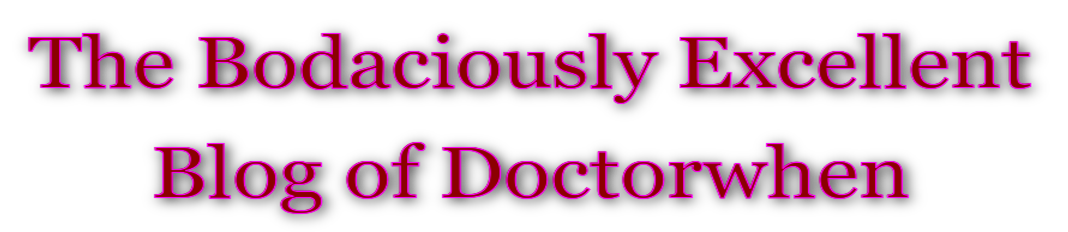# Fractal Art

In mathematics, a fractal is a subset of a Euclidean space for which the Hausdorff dimension strictly exceeds the topological dimension. Here however, we attempt to provide a visual introduction to fractals that does not require any mathematics.

Fractals are infinitely self-similar, iterated, and detailed mathematical constructs having fractional dimension, of which many examples have been formulated and studied in great depth. Fractals are not limited to geometric patterns, but can also describe processes in time. Fractal patterns with various degrees of self-similarity have been rendered or studied in images, structures, sounds, and found in naturetechnologyart, architecture and law. Fractals are of particular relevance in the field of chaos theory, since the graphs of most chaotic processes are fractals.

The fractals in this gallery were created by Dr. Ronald Joe Record who holds a Ph.D. in Mathematics from the University of California. Dr. Record’s research focused on applications of Dynamical Systems Theory, an area of Mathematics popularly known as Chaos Theory.